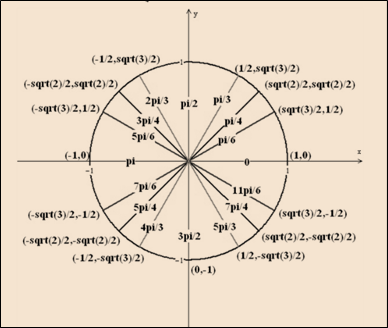# The value of x to satisfy the following inequality equation: − 1 &lt; tan x &lt; 1### Single Variable Calculus: Concepts...

4th Edition
James Stewart
Publisher: Cengage Learning
ISBN: 9781337687805### Single Variable Calculus: Concepts...

4th Edition
James Stewart
Publisher: Cengage Learning
ISBN: 9781337687805

#### Solutions

Chapter C, Problem 35E
To determine

## To calculate: The value of x to satisfy the following inequality equation:   −1<tanx<1

Expert Solution

The values of x for the interval [0,2π] to satisfy eq. 1<tanx<1 are [0xπ4],[3π4x5π4],[7π4x2π] .

### Explanation of Solution

Given information:

1<tanx<1[0,2π]

Calculation:

Know that:

1<tanx<11<sinxcosx<1

That means, for the tangent value of ±1 , the value of sine and cosine value are same with the different signs. It will occurs at only ±12 , angle is odd multiple of π4 .

So, x for the interval [0,2π] are π4,3π4,5π4,7π4 . These angles break into 4 sections as:

In the first quadrant 0<tanx<1 , x value of interval [0xπ4] , it is greater than 1 that means the inequality equation is not satisfied with this range.

In the second quadrant 1<tanx<0 , x value of interval [3π4xπ]

In the third quadrant 0<tanx<1 , x value of interval [πx5π4]

In the fourth quadrant 1<tanx<0 , x value of interval [7π4x2π]

So, the unit circle with co-ordinates (x,y)=(cosx,sinx) and radius 1 :Fig. Unit circle

Therefore, the values of x for the interval [0,2π] to satisfy eq. 1<tanx<1 are [0xπ4],[3π4x5π4],[7π4x2π] .

### Have a homework question?

Subscribe to bartleby learn! Ask subject matter experts 30 homework questions each month. Plus, you’ll have access to millions of step-by-step textbook answers!